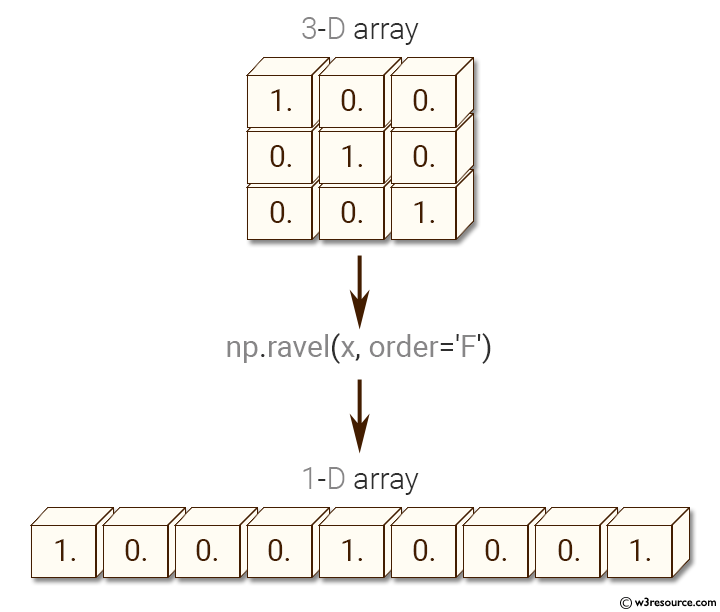﻿ NumPy: Collapse a 3-D array into one dimension array - w3resource# NumPy: Collapse a 3-D array into one dimension array

## NumPy: Array Object Exercise-49 with Solution

Write a NumPy program to collapse a 3-D array into one dimension array.

Pictorial Presentation:Sample Solution:-

Python Code:

``````import numpy as np
x = np.eye(3)
print("3-D array:")
print(x)
f = np.ravel(x, order='F')
print("One dimension array:")
print(f)
```
```

Sample Output:

```3-D array:
[[ 1.  0.  0.]
[ 0.  1.  0.]
[ 0.  0.  1.]]
One dimension array:
[ 1.  0.  0.  0.  1.  0.  0.  0.  1.]
```

Python Code Editor:

Have another way to solve this solution? Contribute your code (and comments) through Disqus.

What is the difficulty level of this exercise?

Test your Python skills with w3resource's quiz

﻿

## Python: Tips of the Day

Set comprehension:

```>>> m = {x ** 2 for x in range(5)}
>>> m
{0, 1, 4, 9, 16}
```